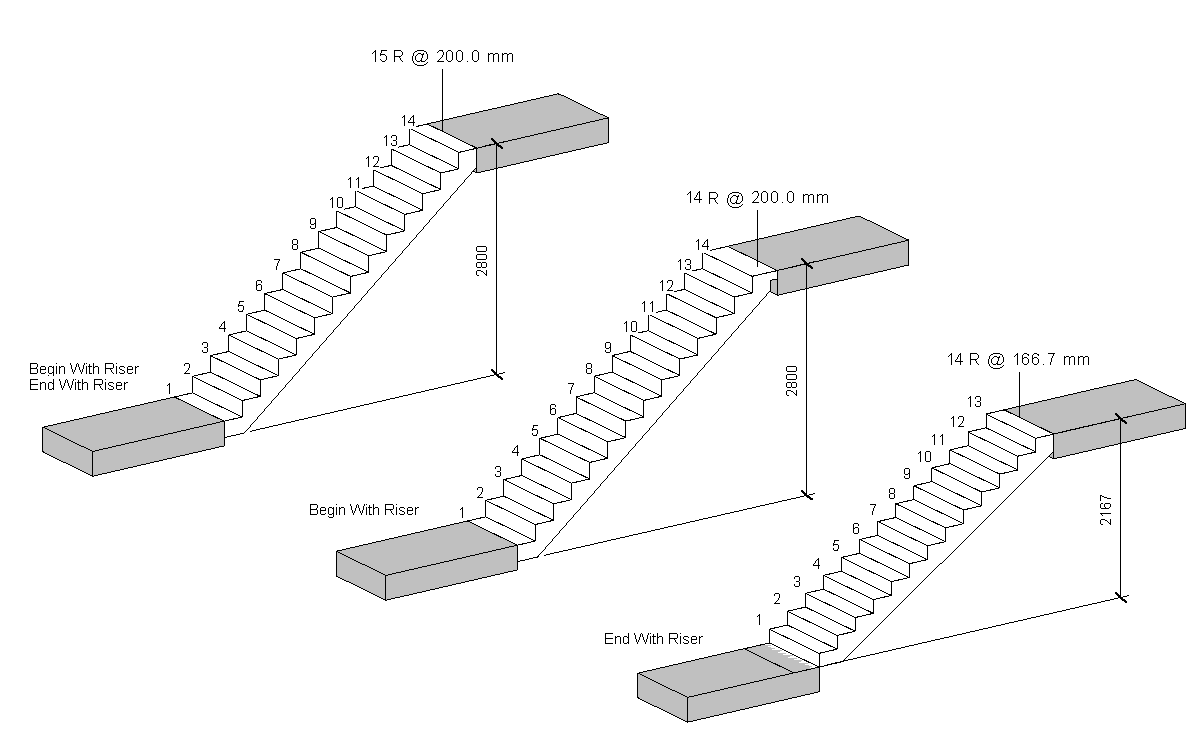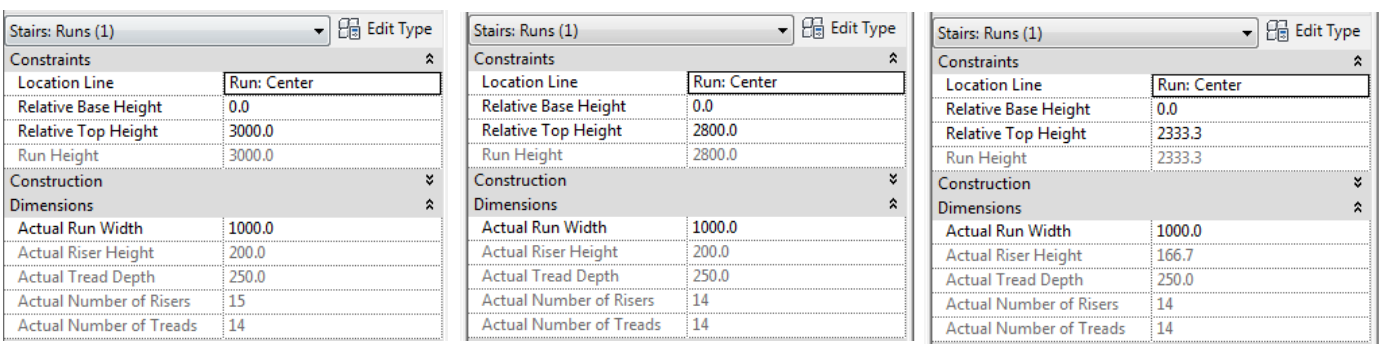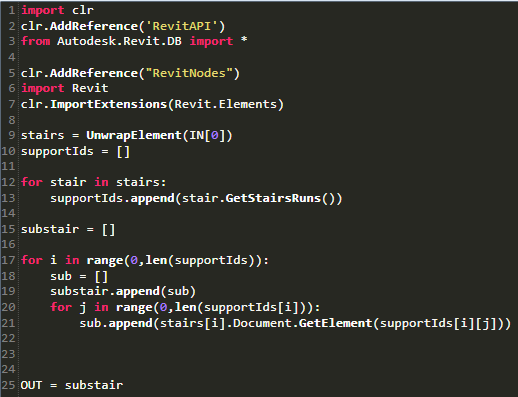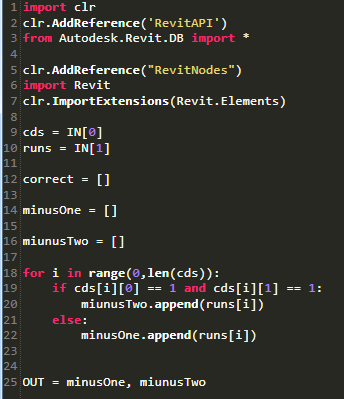Calculate the number of risers in a stair

Stairs in Revit are quite peculiar. The same stair behaves differently if “Begin With Riser” or “End With Riser” are selected.

All these stairs have 13 raisers but Revit counts the risers differently as it does not know where I have drawn the landings (normal structural slabs, separated from the stairs).We can use Dynamo to count the risers and write the result into a new parameter.

Let’s start selecting all the stairs in the Revit model, get the Stair Runs and separate them according to their Begin/End Riser values (both thick or either one or the other tick).Then if the stairs Begin and Ends with a Riser, we can calculate the number of risers using the formula (RUN HEIGHT-2*RISER HEIGHT)/RUN HEIGHT

If the stairs Begin or End with a Riser, we can calculate the number of risers using the formula (RUN HEIGHT-RISER HEIGHT)/RUN HEIGHT

We can the use SetParameterByName to write the result back into Revit.